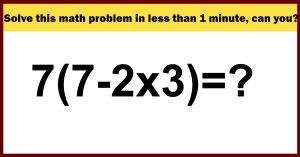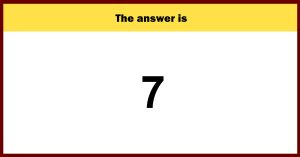Home Lifestyle Solve this math problem in less than 1 minute, can you?

Solve this math problem in less than 1 minute, can you?

Take a look at the math puzzle below and see what you make of it. Also, check the clock before you start. How fast can you do this?

Ready to solve the equation? The time limit is: 1 minuteThe answer will be shown below. Don’t cheat by scrolling down, . Have you reached a solution?

How fast did you get it?

a

n

s

w

e

r

The Solution

Here is how we can break it down: 7(7-2x 3) =?
1- First of all, we need to start with solving the multiplication in brackets 7 (7-6)
2- Then you solve the equation within the brackets 7 (1)
3- Now you can continue with solving the multiplication: 7 x 1 =
4- Now you have the solution to the equation: It’s 7